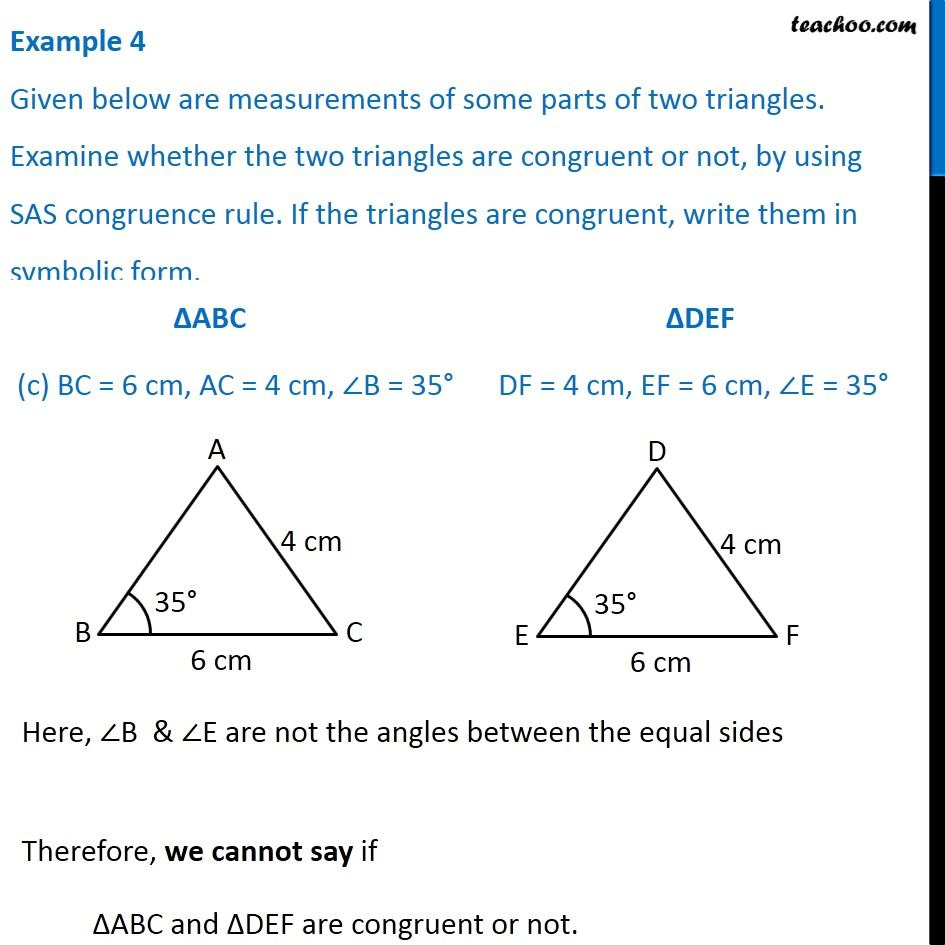Examples

Chapter 7 Class 7 Congruence of Triangles
Serial order wise### Transcript

Example 4 Given below are measurements of some parts of two triangles. Examine whether the two triangles are congruent or not, by using SAS congruence rule. If the triangles are congruent, write them in symbolic form. Here, ∠B & ∠E are not the angles between the equal sides Therefore, we cannot say if ∆ABC and ∆DEF are congruent or not.